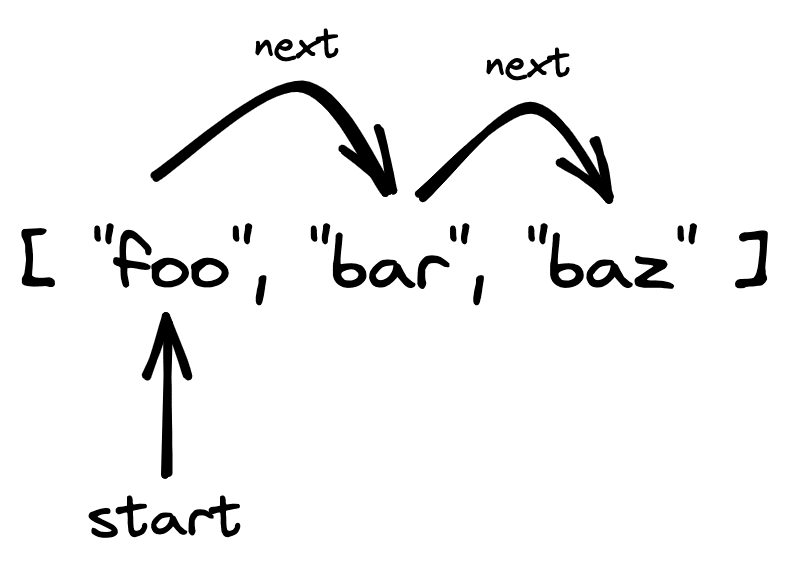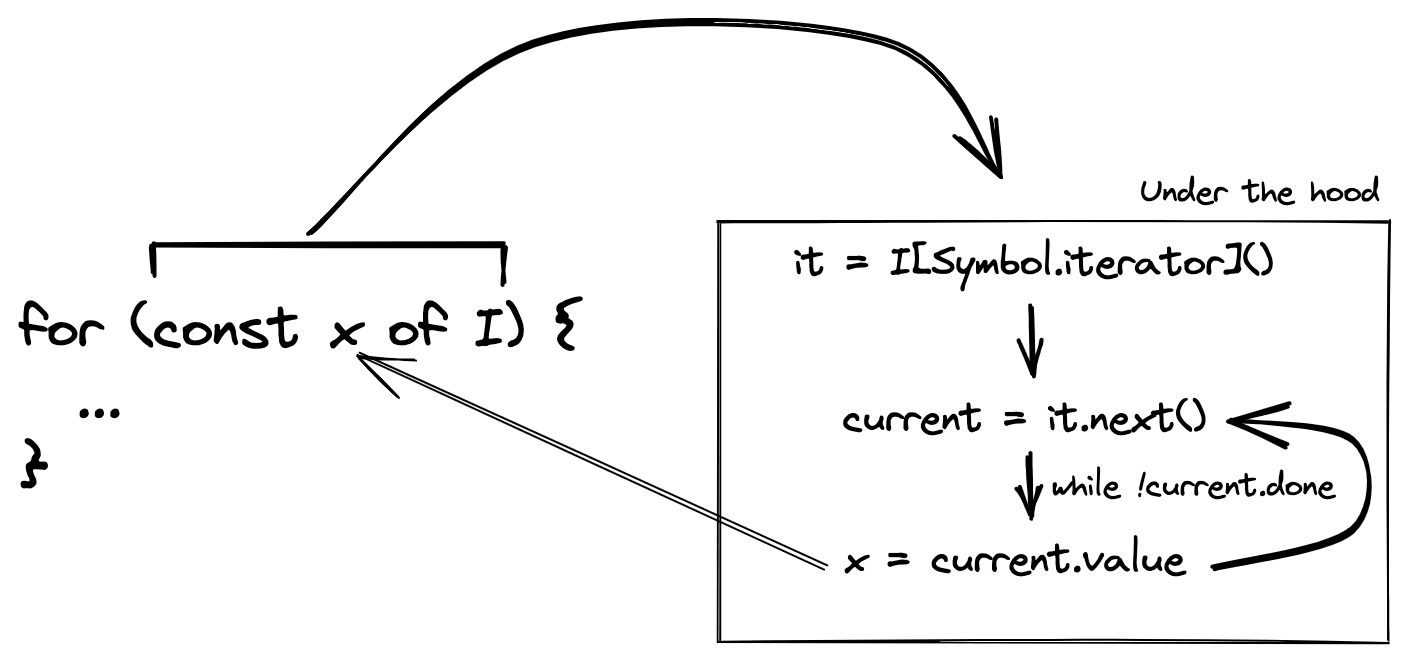# Iterables in JS

July 12, 2022Grant SanderThe JavaScript (JS) language has gone through some changes over the years. One important milestone for the JS language was the formalization and adoption of the ES2015 (aka “ES6”) specification. This milestone brought with it a large handful of lovely language features that us JS developers now take for granted. This handful of features includes block-scoped variables (using `let` and `const` keywords), arrow functions, default parameter values, object destructuring, Promises, and many more.

A perhaps less well-known addition of the ES2015 spec is the addition of the iteration protocols. These protocols allow us JS developers to make use of iterables — a very powerful language feature that you’re likely already using in your day-to-day development, but maybe haven’t given too much thought to! In this post, we’ll explore what iterables are, where you’ve likely already seen them, and how to create your own.

## What is an iterable and what can you do with it?

Conceptually, an “iterable” is an object or value that can be iterated through (or looped over, or stepped through). This is a pretty generic definition because you can conceive of many different data types that you could somehow “step through”.

For example, you might be able to imagine iterating through an array — by visiting each element of the array in order. This is illustrated below.You might also be able to imagine iterating through a string — by visiting each character of the string:It turns out that both arrays and strings are iterables in JS! Iterables in JS can be used in two important ways:

• they can be looped over via a `for...of` loop;
• they can be “spread” via the `...` spread operator.

Here’s a perhaps-familiar example of using an array (which is an iterable data type):

``````const nums = [5, -3, 17];

// Use in for...of loop
for (const num of nums) {
console.log(num); // log: 5, -3, 17
}

Math.max(...nums); // -> 17``````

Since strings are also iterable, you can do something very similar with string values!

``````const str = "Woof";

// Use in for...of loop
for (const char of str) {
// Do something with "W", "o", "o", "f"...
}

[...str]; // -> ['W', 'o', 'o', 'f']``````

Using the spread operator with strings is an interesting alternative to using `String.prototype.split` to split a string into an array of its characters (if you needed to, say, reverse a string).

## The technical definition of an iterable

From a technical perspective, an “iterable” isn’t a specific data type in JS. Rather, it’s a protocol that various data types and objects can implement and the JS engine will treat such values as iterables. The technical requirements for an object to be iterable is as follows:

• The iterable object must have a `@@iterator` method that returns an iterator object. The `@@iterator` key is a symbol that can be accessed via `Symbol.iterator`.
• An iterator object is an object with a `next` method that returns an object of the shape `{ value: T, done: boolean }` that indicates the current value and whether or not the iterator has been exhausted.

This definition is quite technical, but here’s how I like to think about it:

• To loop over an iterable `I`, the JS engine asks for a new iterator from `I` for the engine to step through. It does this by calling the `@@iterator` method of `I`, e.g. `const it = I[Symbol.iterator]()`.
• The JS engine then calls `it.next()` until the the iterator has been exhausted. `it.next()` returns a value of shape `{ value: T, done: boolean }`; the engine gives you access to the `value` field as you’re stepping through the iterator, and uses the `done` field internally to know when to stop calling `it.next()`. Once the engine sees `done:true`, it’ll stop the iteration process right there.

I find this a little easier to think about by writing our own custom implementation of looping over an iterable (such as an array).

``````// A function that takes an iterable, and a function fn,
//  and calls fn on each element of the iterable.
const loopOverIterator = <T>(I: Iterable<T>, fn: (x: T) => void) => {
// Ask the iterable for an iterator to use
const iterator = I[Symbol.iterator]();
// Keep track of current iteration state
let current = iterator.next();

// Keep looping until our iterator has indicated that we're done.
while (!current.done) {
// Use the current value
fn(current.value);
// And ask the iterator to move to the next step
current = iterator.next();
}
};

// Sample usage
loopOverIterator("Howdy", console.log); // log: "H", "o", "w", "d", "y"``````

This is very similar to how a `for...of` loop works under the hood! The diagram below shows how we might think about this when seeing a `for...of` loop, based on our naive implementation above.This section has outlined the technical requirements for a data type or object to be considered an iterable. Let’s check out a concrete example of creating our own custom iterable so we can see what it looks like to implement these technical requirements for an iterable.

## Custom `lineSegment` iterable

### A little bit of setup

In the remainder of this post, we’ll create a custom iterable to represent a line segment. Let’s scratch out a few mathematical details to set the scene. First, check out the diagram below. It’s a line segment between two points `(x1, y1)` and `(x2, y2)`.A line segment is really just a collection of infinitely many points between the two endpoints. Mathematically, we can “parameterize” this line segment by imagining some parameter `t` varying from 0 to 1 and plotting points `(x, y)` where `x = x1 * (1 – t) + x2 * t` and `y = y1 * (1 – t) + y2 * t` as `t` varies.

Now, let’s suppose we want to create a simple representation of such a line segment and we want to make this representation iterable, to simulate moving from the starting point to the ending point. The whole “infinitely many points on the line segment” thing is going to be a bit of a blocker for us in terms of trying to iterate from the starting point to the ending point, so we’ll discretize this a bit and only iterate over `n` equally-spaced points on the line segment, as shown below.### Let’s write some code

Let’s get started with some code. We’ll create a `lineSegment` function that will return a simplified object representation of a line segment:

``````type Point = { x: number; y: number };

export const lineSegment = (start: Point, end: Point, n = 20) => {
return {
start,
end,
n,
};
};``````

This representation is not that sophisticated — we’re just keeping track of the starting and ending points, as well as `n`, the number of points we’ll iterate through as we “iterate” over the line. We can represent a line segment between points `(2, 3)` and `(4, -7)` via `lineSegment({x: 2, y: 3}, {x: 4, y: -7});`.

As it stands, our line segment representation is not iterable (that is, it doesn’t implement the iterable protocol). Let’s make this thing iterable by adding a `Symbol.iterator` method that returns an iterator-compliant object!

``````type Point = { x: number; y: number };

export const lineSegment = (start: Point, end: Point, n = 20) => {
return {
// ...

[Symbol.iterator](): Iterator<Point> {
let i = 0,
t = 0;

return {
next() {
t = i++ / n;
const x = start.x * (1 - t) + end.x * t;
const y = start.y * (1 - t) + end.y * t;
const done = t > 1;

return {
value: { x, y },
done,
};
},
};
},
};
};``````

To traverse a line segment, we envision `t` varying from 0 to 1, but we want to discretize this into `n` equally-spaced sections and therefore we’ll let a discrete integer variable `i` iterate from 0 to `n` and set `t = i / n`. Then, we can use our math formulas to determine corresponding values for `x` and `y` for a given value of `t`.

Our iterator method needs to return an iterator, which is an object with a `next` method that is in charge of incrementing/iterating (our custom incrementing code with `i++` etc.), returning the current value (via `next().value`), and indicating whether or not the iteration has already been completed (via `next().done`).

At this point, our `lineSegment` function is returning an iterable object that can be used with `for...of` loops and the `...` spread operator!

## Generator functions: create iterators with ease

Another important feature added to the JS language spec in ES2015 is generator functions. To keep this post from bloating, I won’t get into the nooks and crannies of generator functions — but you should check out this chapter to learn more about generators. In essence, generator functions allow you to define functions that can pause and resume execution in the middle of the function’s body.

Conveniently, generator functions’ return values comply with the iterator protocol. This entails that generator functions can be used to create iterators, and hence can be used to make an object iterable! Let’s see what this looks like in action.

``````type Point = { x: number; y: number };

export const lineSegment = (start: Point, end: Point, n = 20) => {
return {
// ...

*[Symbol.iterator]() {
let i = 0,
t = 0;

while (i <= n) {
const x = start.x * (1 - t) + end.x * t;
const y = start.y * (1 - t) + end.y * t;
yield { x, y };

t = ++i / n;
}
},
};
};``````

The first thing you should notice in this updated code is that instead of defining a method `[Symbol.iterator]() { ... }`, we’ll define `*[Symbol.iterator]() { ... }` (notice the `*`!). The `*` before the method name indicates that we’re defining a generator function, which will return an iterator and we can use the `yield` keyword to return values for the iterator. Each time a `yield X` call is made, the iterator returns `X` as the value — and once there are no more values to yield, the iterator indicates that it has been exhausted (via `done: true`).

Generators are a powerful construct in certain scenarios, and if you’re in the business of making a struct iterable, they really shine!

## Wrap up

In this post, we went on a short exploration of iterables in JS. We took a bird’s-eye look at what iterables are and what built-in data structures we’re likely already using that are iterable. Then, we got our hands dirty with the technical details of the iterable protocol in JS and how we could implement one ourselves to turn our own custom object into an iterable! If you want to check out another (perhaps more realistic) example of implementing the iterable protocol, check out our `smol-range` package on GitHub.

Although you might not often need to create your own custom iterable data structure, I think it’s worthwhile to have a surface-level understanding of iterables in JS so that you can spot when iterables are being used, and why certain constructs in JS (such as `for...of` loops and `...` spread operator) work the way that they do.